## Example Questions

### Example Question #1 : How To Find The Length Of The Diagonal Of A Trapezoid

Suppose the lengths of the bases of a trapezoid are 1 and 5 respectively.  The altitude of the trapezoid is 4.  What is the diagonal of the trapezoid?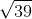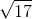Explanation:

The altitude of the trapezoid splits the trapezoid into two right triangles and a rectangle. Choose one of those right triangles. The base length of that right triangle is necessary to solve for the diagonal.

Using the base lengths of the trapezoid, the length of the base of the right triangle can be solved.  The length of the rectangle is 1 unit.  The longer length of the trapezoid base is 5 units.

Since there are 2 right triangles bases that lie on the longer base of the trapezoid, we will assume that their base lengths aresince their lengths are unknown. Combining the lengths of the right triangles and the rectangle, write the equation to solve for the length of the right triangle bases.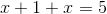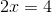The length of each triangular base is 2.

The diagonal of the trapezoid connects from either bottom angle of the trapezoid to the far upper corner of the rectangle.  This diagonal connects to form another right triangle, where the sum of the solved triangular base and the rectangle length is a leg, and the altitude of the trapezoid is another leg.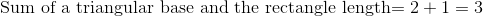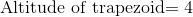Use the Pythagorean Theorem to solve for the diagonal.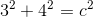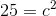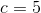### Example Question #2 : How To Find The Length Of The Diagonal Of A Trapezoid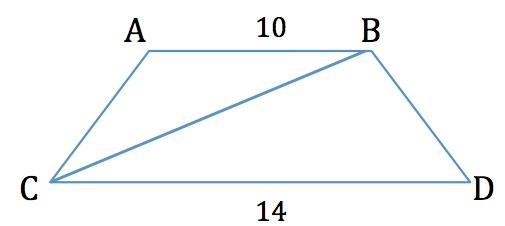If the height of the trapezoid above isunits, what is the length of the diagonal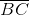?Explanation:

To find the diagonal, we must subtract the top base from the bottom base: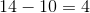This leaves us with 4, which is the sum of the distance to the left and right of the top base. Taking half of that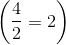gives us the length of the distance to only the left side.

This means that the base of a triangle that includes that diagonal is equal to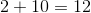.

Since the height is, we can solve this problem either using the Pythagorean Theorum or by remembering that this is a special right triangle (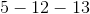triangle). Therefore, the hypotenuse isSee the figure below for clarification: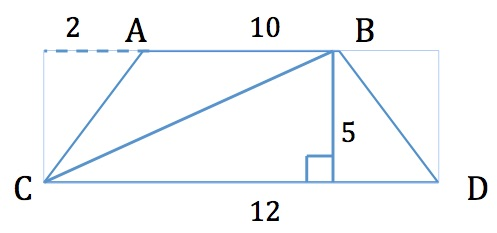### Example Question #3 : How To Find The Length Of The Diagonal Of A Trapezoid

A trapezoid has bases of lengthandand side lengths ofand. What is the upper non-inclusive limit of the trapezoid's diagonal length?In this case,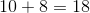, meaning that the diagonal length can go up to but not including.